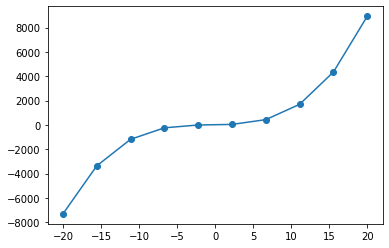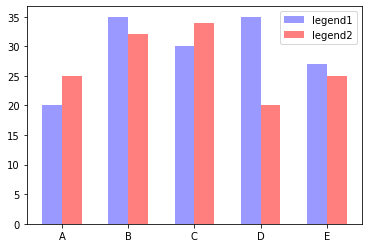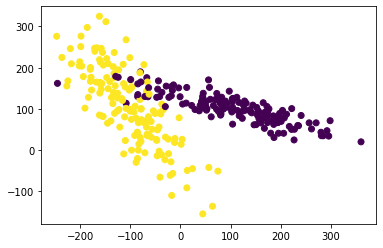# Python机器学习环境的安装和配置¶

## 一、Python环境的安装和配置¶

### 1.1 安装Python环境¶

a. 访问Python官网并下载最新版Python，URL: https://www.python.org/

b. 双击并运行安装，勾选【Add Python 3.8 to PATH】

### 1.2 测试Python环境¶

In :
print("Hello World!")

Hello World!

In :
names = input("请输入各个同学行业名称，行业名称之间用空格间隔（回车结束输入）：")
t = names.split()
d = {}
for c in range(len(t)):
d[t[c]] = d.get(t[c], 0)+1
ls = list(d.items())
ls.sort(key=lambda x: x, reverse=True)
for k in range(len(ls)):
zy, num = ls[k]
print("{}:{}".format(zy, num))

英语:2



### 1.3 安装课程所需要的其他库文件¶

• 安装jieba库

>> pip install jieba

• 安装wordcloud词云库

>> pip install wordcloud

## 2. 标准Python环境安装和配置¶

### 2.1 Python环境的安装与配置¶

• 推荐安装Anaconda，因为Anaconda包含了大量的Python库函数，为了方便后续的使用，建议使用Anaconda封装版的Python。
• URL：https://www.anaconda.com/
• 安装过程较为简单，但后续需要安装各种库时，均需要打开【Anaconda Prompt (Anaconda3)】命令提示行进行安装。

### 2.2 Visual Studio Code (VSCode) 编程环境的安装与配置¶

#### 2.2.1 VSCode的安装¶

• VSCode是当今最流行的集成开发环境，不仅适用于Python，也同样适用于Html+CSS、Javascript及php等Web前端的开发，同时也支持Java、C++、C等程序的开发。
• 最新版本1.39，URL：https://code.visualstudio.com/

#### 2.2.2 VSCode的配置 (可选，但更丰富的插件有利于提高开发效率)¶

VSCode的强大之处来源于各种插件，下面将推荐一些常用及好用的插件。安装时，首先打开左边的【Extensions】标签（4个小方块），或按快捷键【Ctrl+Shift+X】打开插件管理界面。输入插件名称，并点击插件旁的【Install】按钮。

• 自动同步配置
• 插件名：【Settings Sync】
• 使用方法：【Alt+Shift+U/D】（上传/下载）
• Settings Sync插件的使用，需要配合Github使用，此处不再累赘介绍该插件的安装方法，各位可以自行【百度】。
• 配置好【Settings Sync】后，后续的所有安装和配置，基本上就只需要执行一次，将来可以直接使用【Settings Sync】进行同步。同时该插件也可以实现多台计算机配置（及所有插件）的同步。
• 中文界面
• 使用快捷键【Ctrl+Shift+P】打开搜索按钮
• 搜索【Configure Display Language】，选择安装简体中文或直接搜索插件【Chinese (Simplified) Language Pack for Visual Studio Code】
• 安装完成后按照提示重启VSCode，或手动重启VSCode以激活简体中文语言包
• 启用Flake8代码检测
• 点击左下角【Setting】按钮，并搜索【Flake】
• 勾选【Python › Linting: Flake8 Enabled】
• 有用的插件
• Python开发包：Python (Microsoft)
• 突出显示成对的括号：Bracket Pair Colorizer 2+++
• 突出显示缩进：indent-rainbow、 Guides
• 漂亮的文件夹工具包：vscode-icons
• 安装方法：点击左侧【Extensions】
• 有用的命令
• 自动格式化代码：【Alt+Shift+F】
• 其他问题
• 在Python调用第三方库时，pylint无法完成语法检测。
• 解决方法是：打开设置，搜索并编辑【settings.json】；在大括号里增加：【"python.linting.pylintArgs":["--generate-members"]】

### 2.3 JupyterLab 编程环境的安装与配置¶

#### 2.3.1 JupyterLab的安装¶

• 打开【Anaconda Prompt (Anaconda3)】并执行以下语句

>> conda install jupyterlab

• 更新JupyterLab内核

>> conda update jupyter_core jupyter_client

#### 2.3.2 JupyterLab的配置 (可选)¶

• 修改JupyterLab的默认路径

• 生成配置文件

>> jupyter notebook --generate-config

• 编辑配置文件

• 打开【C:\Users\计算机的用户名.jupyter\jupyter_notebook_config.py】
• 修改字段【c.NotebookApp.notebook_dir】为指定路径
• 其中【C:\Users\计算机的用户名】为Anaconda的默认路径，可以通过启动【Anaconda Prompt (Anaconda3)】查看默认地址。
• 设置快捷打开JupyterLab

默认情况下，打开JupyterLab，需要先打开【Anaconda Prompt (Anaconda3)】，然后在命令行中输入【jupyter lab】，此处提供几种基于批处理(*.bat)的快速打开JupyterLab的方法。

a. 右键点击【Anaconda Prompt (Anaconda3)】的属性，查看【目标】字段，拷贝“/K” 后面的内容。

b. 新建一个文本文件，输入以下字段 方法一：使用Windows命令行启用 （方法一可免去设置默认路径）

C:\ProgramData\Anaconda3\Scripts\jupyter-lab.exe  D:\CloudStation\MyWebsites\Teaching\


C:\Users\oxy\Anaconda3\Scripts\jupyter-lab.exe  D:\CloudStation\MyWebsites\Teaching


CALL C:\ProgramData\Anaconda3\Scripts\activate.bat C:\ProgramData\Anaconda3
jupyter lab


c. 另存为JupyterLab.bat

d. 使用时，只需要双击该批处理(*.bat)文件即可

## 二、 机器学习必需库的安装和测试¶

基于Anaconda开发包安装的Python，下列的5个库都不需要进行额外安装，基于官方版的Python需要额外进行安装，请各位自行查阅安装方法。

### 1. Numpy 基础科学计算库¶

Numpy是Python中最基础的科学计算库，它的功能主要包括高位数组（Array）计算、线性代数计算、傅里叶变换以及产生伪随机数等。Numpy是机器学习库scikit-learn的重要组成部分，因为机器学习库scikit-learn主要依赖于数组形式的数据来进行处理。

【知识点】[Numpy基础科学库极简使用说明](functions/reshape.ipynb)

以下代码用于测试和生成一个数组。

In :
# 使用import关键字引入numpy库，为了简便使用缩写 “np”来表示numpy库。
import numpy as np
# 定义一个变量 i, 用于保存数组
i = np.array([[12,34,56],[78,90,11]])

In :
# 输出变量 i
print("i = \n{}".format(i))

i =
[[12 34 56]
[78 90 11]]


### 2. Scipy 科学计算工具集¶

Scipy是Python中用于进行科学计算的工具集，它可以实现计算机统计学分布、信号处理、线性代数方程等功能。在机器学习中，稀疏矩阵的使用非常频繁，Scipy库中的sparse函数可以用来生成这种稀疏矩阵。稀疏矩阵用于存储那些大部分数值为0的np数组。以下代码用使用sparse()函数生成和测试稀疏矩阵。

In :
# 对scipy的使用需要利用from关键字来引用其内部的子库
import numpy as np
from scipy import sparse

# 使用numpy的eye()函数生成一个6行6列的对角矩阵
# 矩阵中对角线上的元素值为 1，其余元素为 0
matrix = np.eye(6)

# 将np数组转化为 CSR格式的Scipy稀疏矩阵 (sparse matrix)
sparse_matrix = sparse.csr_matrix(matrix)

In :
# 输出对角矩阵
print("对角矩阵：\n{}".format(matrix))

对角矩阵：
[[1. 0. 0. 0. 0. 0.]
[0. 1. 0. 0. 0. 0.]
[0. 0. 1. 0. 0. 0.]
[0. 0. 0. 1. 0. 0.]
[0. 0. 0. 0. 1. 0.]
[0. 0. 0. 0. 0. 1.]]

In :
# 输出CSR格式的稀疏矩阵
print("CSR格式的稀疏矩阵：\n{}".format(sparse_matrix))

CSR格式的稀疏矩阵：
(0, 0)	1.0
(1, 1)	1.0
(2, 2)	1.0
(3, 3)	1.0
(4, 4)	1.0
(5, 5)	1.0


### 3. Pandas 数据分析工具¶

Pandas是Python中进行数据分析的库，它具有以下功能

• 生成类似Excel表格式的数据表，并对数据进行修改操作；
• 从不同的数据源中获取数据，例如：SQL Server, Excel表格, CSV文件, Oracle等；
• 在不同的列中使用不同的数据类型，例如：整型，浮点型，字符串型等。
• 更多信息请参考“Pandas中文网”，URL：https://www.pypandas.cn/
In :
# 使用import关键字引入pandas库，为了简便使用缩写 “pd”来表示pandas库。
import pandas as pd

# 使用字典数据类型创建一个数据表，并用pandas库的DataFrame数据结构进行显示
data = {"姓名":["张飞","赵云","夏侯惇","太史慈"],
"归属国":["蜀国","蜀国","魏国","吴国"],
"年龄":["33","28","32","30"],
"武力值":["98","97","94","92"],
"战斗力":["100","101","98","97"]
}

data_frame = pd.DataFrame(data) # 将字典数据类型转换成pandas数据类型
display(data_frame)
# 值得注意的是display是Jupyter-iPython内置函数，所以在VS中是不起作用。


0 张飞 蜀国 33 98 100
1 赵云 蜀国 28 97 101
2 夏侯惇 魏国 32 94 98
3 太史慈 吴国 30 92 97
• 基于数值模式的字典

In :
# 使用import关键字引入pandas库，为了简便使用缩写 “pd”来表示pandas库。
import pandas as pd

# 使用字典数据类型创建一个数据表，并用pandas库的DataFrame数据结构进行显示
data = {"姓名":["张飞","赵云","夏侯惇","太史慈"],
"归属国":["蜀国","蜀国","魏国","吴国"],
"年龄":[33,28,32,30],
"武力值":[98,97,94,92],
"战斗力":[100,101,98,97]
}

data_frame = pd.DataFrame(data) # 将字典数据类型转换成pandas数据类型
display(data_frame)
# 值得注意的是display是Jupyter-iPython内置函数，所以在VS中是不起作用。


0 张飞 蜀国 33 98 100
1 赵云 蜀国 28 97 101
2 夏侯惇 魏国 32 94 98
3 太史慈 吴国 30 92 97
• 如果想要把一些数据段进行排除，可以使用查询语句来实现。例如，不显示“魏国”的武将信息。
In :
# 使用 “不等于 !=” 操作符排除字段中包含特定值的数据
display(data_frame[data_frame.归属国 != "魏国"])


0 张飞 蜀国 33 98 100
1 赵云 蜀国 28 97 101
3 太史慈 吴国 30 92 97
• 尝试输出战斗力大于97的武将信息
In :
display(data_frame[data_frame.战斗力 > "97"])


2 夏侯惇 魏国 32 94 98

In :
display(data_frame[data_frame.战斗力 > 97])


0 张飞 蜀国 33 98 100
1 赵云 蜀国 28 97 101
2 夏侯惇 魏国 32 94 98

### 4. Matplotlib 绘图库¶

matplotlib是Python中最重要的绘图库，它可以生成出版质量级别的图形，包括折线图、散点图、直方图等。

In :
# 通过inline指令，实现在Jupyter中的实时绘图功能
%matplotlib inline

# 1. 使用import关键字引入matplotlib库，为了简便使用缩写 “plt”来表示matplotlib库。
import matplotlib.pyplot as plt
import numpy as np

# 使用linspace()函数生成一个-20到20，元素个数为10的等差数列。
# 令数列中的值为 x, 并根据表达式计算对应的 y值。
x = np.linspace(-20, 20, 10)
y = x**3 + 2*x**2 + 6*x + 5

#使用plot()函数绘制出曲线图
plt.plot(x, y, marker = "o")

Out:
[<matplotlib.lines.Line2D at 0x1dc34fe5a88>]In :
print("x={}".format(x))
print("y={}".format(y))

x=[-20.         -15.55555556 -11.11111111  -6.66666667  -2.22222222
2.22222222   6.66666667  11.11111111  15.55555556  20.        ]
y=[-7315.         -3368.4430727  -1186.4951989   -242.40740741
-9.43072702    39.18381344   430.18518519  1690.3223594
4346.34430727  8925.        ]


In :
# 1. 引入库
# 通过inline指令，实现在Jupyter中的实时绘图功能
%matplotlib inline
import matplotlib.pyplot as plt

# 2. 指定坐标轴上的值
plt.figure(1)
x_index = np.arange(5)   #柱的索引
x_data = ('A', 'B', 'C', 'D', 'E')
y1_data = (20, 35, 30, 35, 27)
y2_data = (25, 32, 34, 20, 25)
bar_width = 0.3   #定义一个数字代表每个独立柱的宽度

# 3. 绘图
# 使用 bar()函数定义柱状图的各个参数，依次包括：左偏移、高度、柱宽、透明度、颜色、图例
# 关于左偏移，不用关心每根柱的中心不中心，因为只要把刻度线设置在柱的中间就可以了
rects1 = plt.bar(x_index, y1_data, width=bar_width,alpha=0.4, color='b',label='legend1')
rects2 = plt.bar(x_index + bar_width, y2_data, width=bar_width,alpha=0.5,color='r',label='legend2')

# 4. 优化图形
# 使用 xticks() 函数设置x轴的刻度线
plt.xticks(x_index + bar_width/2, x_data)
plt.legend() #显示图例
plt.show()### 5. scikit-learn 机器学习库¶

scikit-learn是Python中最重要的机器学习模块之一。它基于Scipy库，在不同的领域中已经发展出大量基于Scipy的工具包，它们被统一称为Scikits，其中最著名的一个分支就是scikit-learn。它包含众多的机器学习算法，主要分为六大类：分类、回归、聚类、数据降维、模型选择和数据预处理。下列给出一个使用scikit-learn进行分类的简单例子。在下例中会随机生成包含300个具有两种属性数据的数据集，然后利用简单的SVM分类器实现分类。

1. 加载分类模型和可视化模块所需要的库文件
In :
# 载入基础科学计算库 numpy
import numpy as np
# 载入可视化数据的模块 matplotlib
import matplotlib.pyplot as plt

# 从scikit-learn 库中载入预处理模块, 数据生成模块, 数据分割模块(划分为
# 训练集和测试集)和 支持向量机SVM的Support Vector Classifier分类模块
from sklearn.datasets.samples_generator import make_classification
from sklearn import preprocessing
from sklearn.model_selection import train_test_split
from sklearn.svm import SVC

1. 生成数据集
In :
# 生成300个具有2种属性的数据
X, y = make_classification(n_samples=300, n_features=2,
n_redundant=0, n_informative=2,
random_state=22, n_clusters_per_class=1,
scale=100)

1. 可视化数据并计算分类精度
In :
#可视化数据
plt.scatter(X[:, 0], X[:, 1], c=y)
plt.show()

# 实现数据的正则化，可以有效提高分类精度
X = preprocessing.scale(X)

# 使用 train_test_split() 函数，将样本分割为 train训练集和 test测试集，
# 其中测试集数量为 30%
X_train, X_test, y_train, y_test = train_test_split(X, y, test_size=0.3)

# 定义SVC的核函数
clf = SVC(gamma = "auto")
# 使用fit()函数对模型进行训练
clf.fit(X_train, y_train)
# 使用 test测试集输出测试准确率
print(clf.score(X_test, y_test))0.9666666666666667